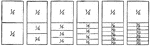### Fraction Comparisons

Rectangles comparing fraction values to one half: 1/4, 1/6, 1/8, 1/10, and 1/12.### Fraction Pie Divided into Eighths

A circle divided into eighths.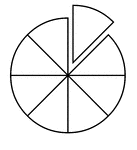### Fraction Pie Divided into Eighths

A circle divided into eighths with one eighth separated.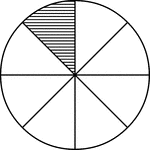### Fraction Pie Divided into Eighths

A circle divided into eighths with one eighth shaded.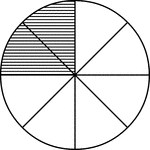### Fraction Pie Divided into Eighths

A circle divided into eighths with two eighths shaded.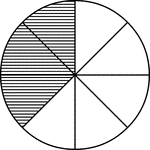### Fraction Pie Divided into Eighths

A circle divided into eighths with three eighths shaded.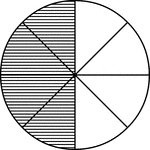### Fraction Pie Divided into Eighths

A circle divided into eighths with four eighths shaded.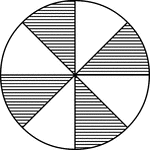### Fraction Pie Divided into Eighths

A circle divided into eighths with four eighths shaded.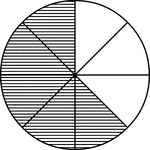### Fraction Pie Divided into Eighths

A circle divided into eighths with five eighths shaded.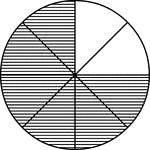### Fraction Pie Divided into Eighths

A circle divided into eighths with six eighths shaded.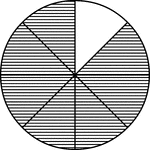### Fraction Pie Divided into Eighths

A circle divided into eighths with seven eighths shaded.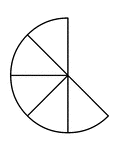### Five Eighths of a Fraction Pie

Five eighths of a circle.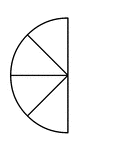### Four Eighths of a Fraction Pie

Four eighths of a circle.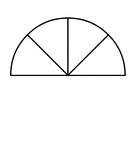### Four Eighths of a Fraction Pie

Four eighths of a circle.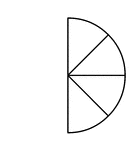### Four Eighths of a Fraction Pie

Four eighths of a circle.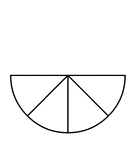### Four Eighths of a Fraction Pie

Four eighths of a circle.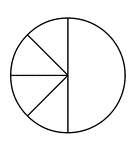### Four Eighths and One Half of a Pie Fraction

A circle subdivided into four eighths and one half.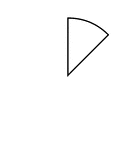### One Eighth of a Fraction Pie

One eighth of a circle.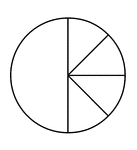### One Half and Four Eighths of a Pie Fraction

A circle subdivided into one half and four eighths.### One Quarter, Two Eighths, and One Half of a Pie Fraction

A circle subdivided into one quarter, two eighths, and one half.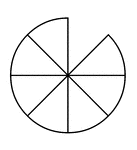### Seven Eighths of a Fraction Pie

Seven eighths of a circle.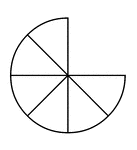### Six Eighths of a Fraction Pie

Six eighths of a circle.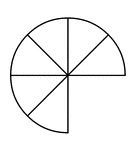### Six Eighths of a Fraction Pie

Six eighths of a circle.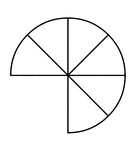### Six Eighths of a Fraction Pie

Six eighths of a circle.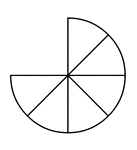### Six Eighths of a Fraction Pie

Six eighths of a circle.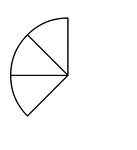### Three Eighths of a Fraction Pie

Three eighths of a circle.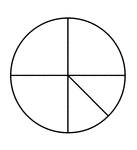### Three Quarters and Two Eighths of a Pie Fraction

A circle subdivided into three quarters and two eighths.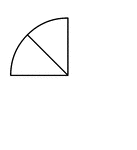### Two Eighths of a Fraction Pie

Two eighths of a circle.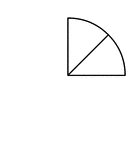### Two Eighths of a Fraction Pie

Two eighths of a circle.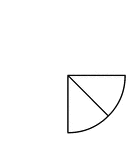### Two Eighths of a Fraction Pie

Two eighths of a circle.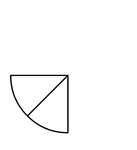### Two Eighths of a Fraction Pie

Two eighths of a circle.